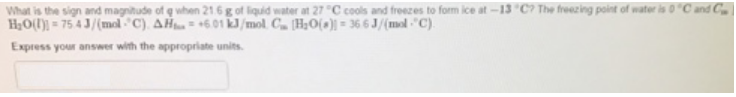Chemistry Calorimetry Solution: What is the sign and magnitude of q when 21.6 g of...

# Solution: What is the sign and magnitude of q when 21.6 g of liquid water at 27 °C cools and freezes to form ice at -13 °C? The freezing point of water is 0°C and Cw H2O(l) = 75.4 J/mol°C.  Express your answer with the appropriate units.

Problem

What is the sign and magnitude of q when 21.6 g of liquid water at 27 °C cools and freezes to form ice at -13 °C? The freezing point of water is 0°C and Cw H2O(l) = 75.4 J/mol°C.  Express your answer with the appropriate units.View Complete Written Solution